V1

2022/10/28阅读：44主题：默认主题

# 0.信息抽取定义以及难点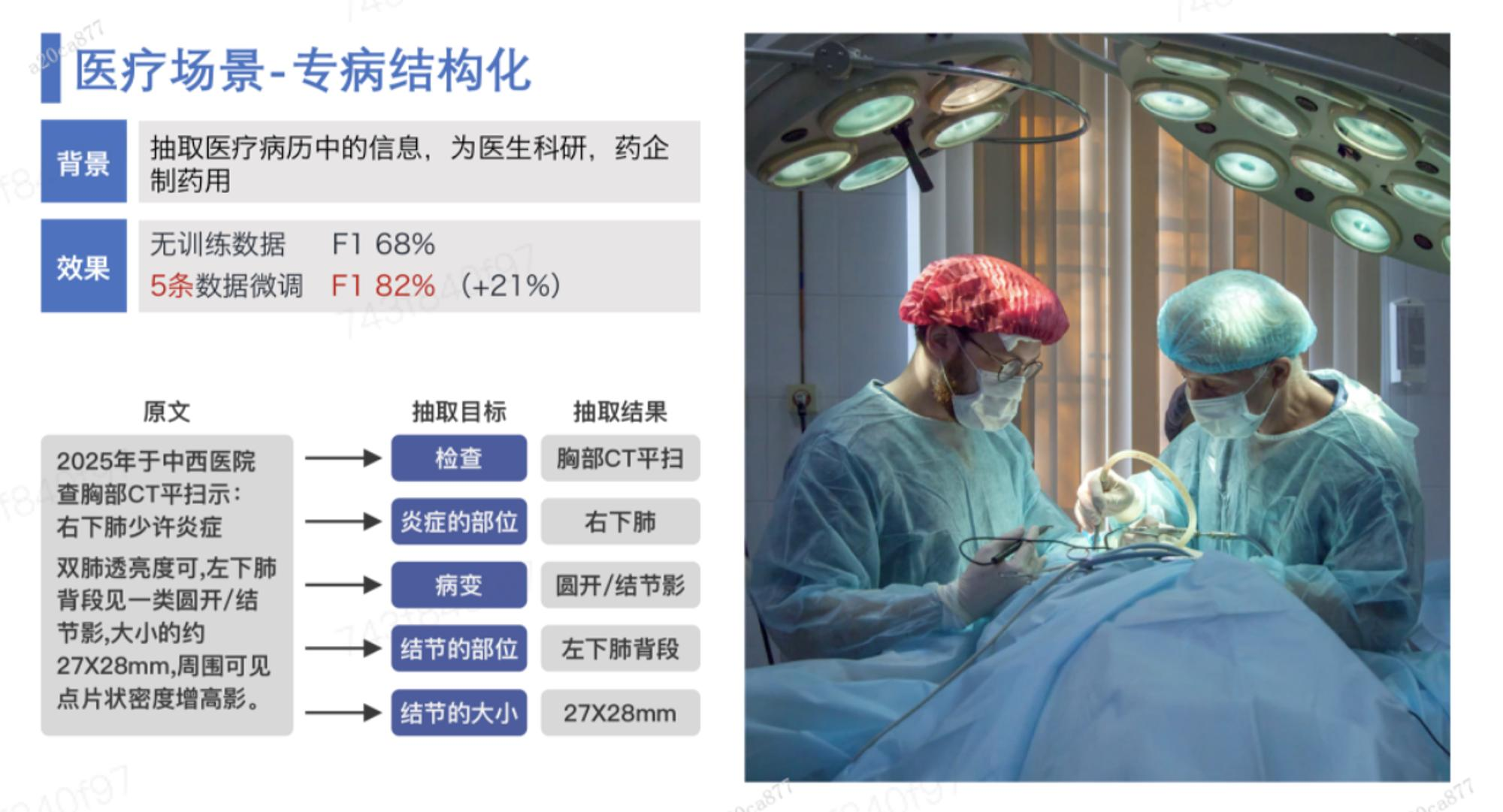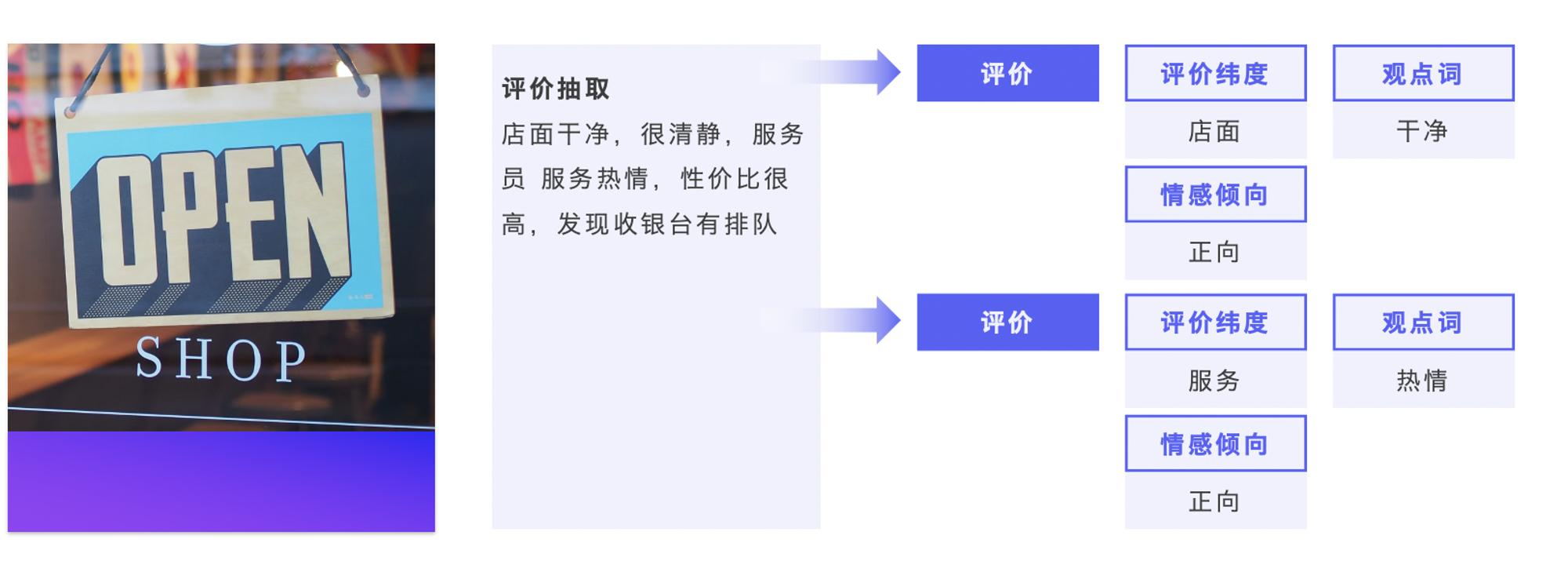• 需求跨领域跨任务：领域之间知识迁移难度高，如通用领域知识很难迁移到垂类领域，垂类领域之间的知识很难相互迁移；存在实体、关系、事件等不同的信息抽取任务需求。
• 定制化程度高：针对实体、关系、事件等不同的信息抽取任务，需要开发不同的模型，开发成本和机器资源消耗都很大。
• 训练数据无或很少：部分领域数据稀缺，难以获取，且领域专业性使得数据标注门槛高。

``! pip install --upgrade paddlenlp! pip show paddlenlp``

### 人力资源入职证明信息抽取

``from paddlenlp import Taskflow schema = ['姓名', '毕业院校', '职位', '月收入', '身体状况']ie = Taskflow('information_extraction', schema=schema)``
``schema = ['姓名', '毕业院校', '职位', '月收入', '身体状况']ie.set_schema(schema)ie('兹证明凌霄为本单位职工，已连续在我单位工作5 年。学历为嘉利顿大学毕业，目前在我单位担任总经理助理  职位。近一年内该员工在我单位平均月收入（税后）为  12000 元。该职工身体状况良好。本单位仅此承诺上述表述是正确的，真实的。')``
``[{'姓名': [{'text': '凌霄',    'start': 3,    'end': 5,    'probability': 0.9042383385504706}],  '毕业院校': [{'text': '嘉利顿大学',    'start': 28,    'end': 33,    'probability': 0.9927952662605009}],  '职位': [{'text': '总经理助理',    'start': 44,    'end': 49,    'probability': 0.9922470268350594}],  '月收入': [{'text': '12000 元',    'start': 77,    'end': 84,    'probability': 0.9788556518998917}],  '身体状况': [{'text': '良好',    'start': 92,    'end': 94,    'probability': 0.9939678710475306}]}]``
``# Jupyter Notebook默认做了格式化输出，如果使用其他代码编辑器，可以使用Python原生包pprint进行格式化输出from pprint import pprintpprint(ie('兹证明凌霄为本单位职工，已连续在我单位工作5 年。学历为嘉利顿大学毕业，目前在我单位担任总经理助理  职位。近一年内该员工在我单位平均月收入（税后）为  12000 元。该职工身体状况良好。本单位仅此承诺上述表述是正确的，真实的。'))``

### 医疗病理分析

``schema = ['肿瘤部位', '肿瘤大小']ie.set_schema(schema)ie('胃印戒细胞癌，肿瘤主要位于胃窦体部，大小6*2cm，癌组织侵及胃壁浆膜层，并侵犯血管和神经。')``
``[{'肿瘤部位': [{'text': '胃窦体部',    'start': 13,    'end': 17,    'probability': 0.9601818899487213}],  '肿瘤大小': [{'text': '6*2cm',    'start': 20,    'end': 25,    'probability': 0.9670914301489972}]}]``

``# 实体抽取schema = ['时间', '赛手', '赛事名称']ie.set_schema(schema)ie('2月8日上午北京冬奥会自由式滑雪女子大跳台决赛中中国选手谷爱凌以188.25分获得金牌！')``
``[{'时间': [{'text': '2月8日上午',    'start': 0,    'end': 6,    'probability': 0.9857379716035553}],  '赛手': [{'text': '中国选手谷爱凌',    'start': 24,    'end': 31,    'probability': 0.7232891682586384}],  '赛事名称': [{'text': '北京冬奥会自由式滑雪女子大跳台决赛',    'start': 6,    'end': 23,    'probability': 0.8503080086948529}]}]``
``# 关系抽取schema = {'歌曲名称': ['歌手', '所属专辑']}  ie.set_schema(schema)ie('《告别了》是孙耀威在专辑爱的故事里面的歌曲')``
``[{'歌曲名称': [{'text': '告别了',    'start': 1,    'end': 4,    'probability': 0.629614912348881,    'relations': {'歌手': [{'text': '孙耀威',       'start': 6,       'end': 9,       'probability': 0.9988381005599081}],     '所属专辑': [{'text': '爱的故事',       'start': 12,       'end': 16,       'probability': 0.9968462078543183}]}},   {'text': '爱的故事',    'start': 12,    'end': 16,    'probability': 0.28168707817316374,    'relations': {'歌手': [{'text': '孙耀威',       'start': 6,       'end': 9,       'probability': 0.9951415104192272}]}}]}]``
``# 事件抽取schema = {'地震触发词': ['地震强度', '时间', '震中位置', '震源深度']}  # 事件需要通过xxx触发词来选择触发词ie.set_schema(schema)ie('中国地震台网正式测定：5月16日06时08分在云南临沧市凤庆县(北纬24.34度，东经99.98度)发生3.5级地震，震源深度10千米。')``
``[{'地震触发词': [{'text': '地震',    'start': 56,    'end': 58,    'probability': 0.9977425555988333,    'relations': {'地震强度': [{'text': '3.5级',       'start': 52,       'end': 56,       'probability': 0.998080217831891}],     '时间': [{'text': '5月16日06时08分',       'start': 11,       'end': 22,       'probability': 0.9853299772936026}],     '震中位置': [{'text': '云南临沧市凤庆县(北纬24.34度，东经99.98度)',       'start': 23,       'end': 50,       'probability': 0.7874014521275967}],     '震源深度': [{'text': '10千米',       'start': 63,       'end': 67,       'probability': 0.9937974422968665}]}}]}]``
``# 情感倾向分类schema = '情感倾向[正向，负向]' # 分类任务需要[]来设置分类的labelie.set_schema(schema) ie('这个产品用起来真的很流畅，我非常喜欢')``
``[{'情感倾向[正向，负向]': [{'text': '正向', 'probability': 0.9990024058203417}]}]``
``# 评价抽取schema = {'评价维度': ['观点词', '情感倾向[正向，负向]']}  # 评价抽取的schema是固定的，后续直接按照这个schema进行观点抽取ie.set_schema(schema) # Reset schemaie('地址不错，服务一般，设施陈旧')``
``[{'评价维度': [{'text': '地址',    'start': 0,    'end': 2,    'probability': 0.9888139270606509,    'relations': {'观点词': [{'text': '不错',       'start': 2,       'end': 4,       'probability': 0.9927845886615216}],     '情感倾向[正向，负向]': [{'text': '正向', 'probability': 0.998228967796706}]}},   {'text': '设施',    'start': 10,    'end': 12,    'probability': 0.9588298547520608,    'relations': {'观点词': [{'text': '陈旧',       'start': 12,       'end': 14,       'probability': 0.928675281256794}],     '情感倾向[正向，负向]': [{'text': '负向', 'probability': 0.9949388606013692}]}},   {'text': '服务',    'start': 5,    'end': 7,    'probability': 0.9592857070501211,    'relations': {'观点词': [{'text': '一般',       'start': 7,       'end': 9,       'probability': 0.9949359182521675}],     '情感倾向[正向，负向]': [{'text': '负向', 'probability': 0.9952498258302498}]}}]}]``
``# 跨任务跨领域抽取schema = ['寺庙', {'丈夫': '妻子'}]  # 抽取的任务中包含了实体抽取和关系抽取ie.set_schema(schema)ie('李治即位后，让身在感业寺的武则天续起头发，重新纳入后宫。')``
``[{'寺庙': [{'text': '感业寺',    'start': 9,    'end': 12,    'probability': 0.9888581774497425}],  '丈夫': [{'text': '李治',    'start': 0,    'end': 2,    'probability': 0.989690572797457,    'relations': {'妻子': [{'text': '武则天',       'start': 13,       'end': 16,       'probability': 0.9987625986790256}]}}]}]``

### 1.4.1. 调整batch_size提升预测效率

``from paddlenlp import Taskflowschema = ['费用']ie.set_schema(schema)ie = Taskflow('information_extraction', schema=schema, batch_size=2) #资源不充裕情况，batch_size设置小点，利用率增加。。ie(['二十号21点49分打车回家46块钱', '8月3号往返机场交通费110元', '2019年10月17日22点18分回家打车46元', '三月三0号23点10分加班打车21元'])``
``[{'费用': [{'text': '46块钱',    'start': 13,    'end': 17,    'probability': 0.9781786110574338}]}, {'费用': [{'text': '110元',    'start': 11,    'end': 15,    'probability': 0.9504088995163151}]}, {'费用': [{'text': '46元',    'start': 21,    'end': 24,    'probability': 0.9753814247531167}]}, {'费用': [{'text': '21元',    'start': 15,    'end': 18,    'probability': 0.9761294626311425}]}]``

### 1.4.2. 使用UIE-Tiny模型来加快模型预测速度

``from paddlenlp import Taskflowschema = ['费用']ie.set_schema(schema)ie = Taskflow('information_extraction', schema=schema, batch_size=2, model='uie-tiny') #ie(['二十号21点49分打车回家46块钱', '8月3号往返机场交通费110元', '2019年10月17日22点18分回家打车46元', '三月三0号23点10分加班打车21元'])``
``[{'费用': [{'text': '46块钱',    'start': 13,    'end': 17,    'probability': 0.8945340489542026}]}, {'费用': [{'text': '110元',    'start': 11,    'end': 15,    'probability': 0.9757676375014448}]}, {'费用': [{'text': '46元',    'start': 21,    'end': 24,    'probability': 0.860397941604333}]}, {'费用': [{'text': '21元',    'start': 15,    'end': 18,    'probability': 0.8595131018474689}]}]``

# 2.小样本提升UIE效果

Taskflow中的UIE基线版本我们是通过大量的有标签样本进行训练，但是UIE抽取的效果面对部分子领域的效果也不是令人满意，UIE可以通过小样本就可以快速提升效果。 为什么UIE可以通过小样本来提升效果呢？UIE的建模方式主要是通过 `Prompt` 方式来建模， `Prompt` 在小样本上进行微调效果非常有效，下面我们通过一个具体的case 来展示UIE微调的效果。## 2.1语音报销工单信息抽取

### 2. 挑战

``ie.set_schema(['时间', '出发地', '目的地', '费用'])ie('10月16日高铁从杭州到上海南站车次d5414共48元')  # 无法准确抽取出发地、目的地``
``[{'时间': [{'text': '10月16日',    'start': 0,    'end': 6,    'probability': 0.9552445817793149}],  '出发地': [{'text': '杭州',    'start': 9,    'end': 11,    'probability': 0.5713024802221334}],  '费用': [{'text': '48元',    'start': 24,    'end': 27,    'probability': 0.8932524634666485}]}]``

## 2.2 标注数据

Step 1. 本地安装doccano（请勿在AI Studio内部运行，本地测试环境python=3.8）

`\$ pip install doccano`

Step 2. 初始化数据库和账户（用户名和密码可替换为自定义值）

`\$ doccano init`

`\$ doccano createuser --username my_admin_name --password my_password`

Step 3. 启动doccano

• 在一个窗口启动doccano的WebServer，保持窗口

`\$ doccano webserver --port 8000`

• 在另一个窗口启动doccano的任务队列

`\$ doccano task`

Step 4. 运行doccano来标注实体和关系

• 打开浏览器（推荐Chrome），在地址栏中输入`http://127.0.0.1:8000/`后回车即得以下界面。
• 登陆账户。点击右上角的`LOGIN`，输入Step 2中设置的用户名和密码登陆。

• 创建项目。点击左上角的`CREATE`，跳转至以下界面。

• 勾选序列标注（`Sequence Labeling`
• 填写项目名称（`Project name`）等必要信息
• 勾选允许实体重叠（`Allow overlapping entity`）、使用关系标注（`Use relation labeling`
• 创建完成后，项目首页视频提供了从数据导入到导出的七个步骤的详细说明。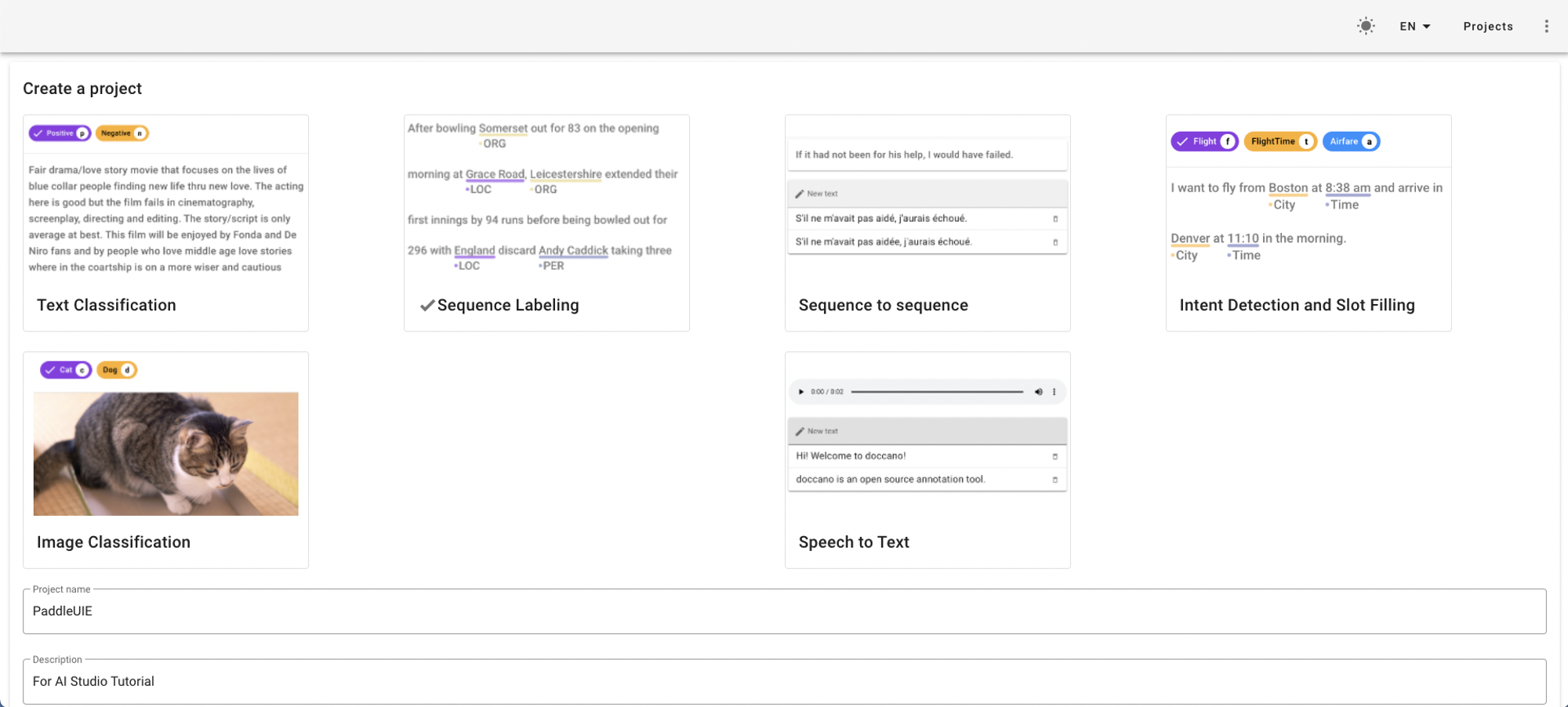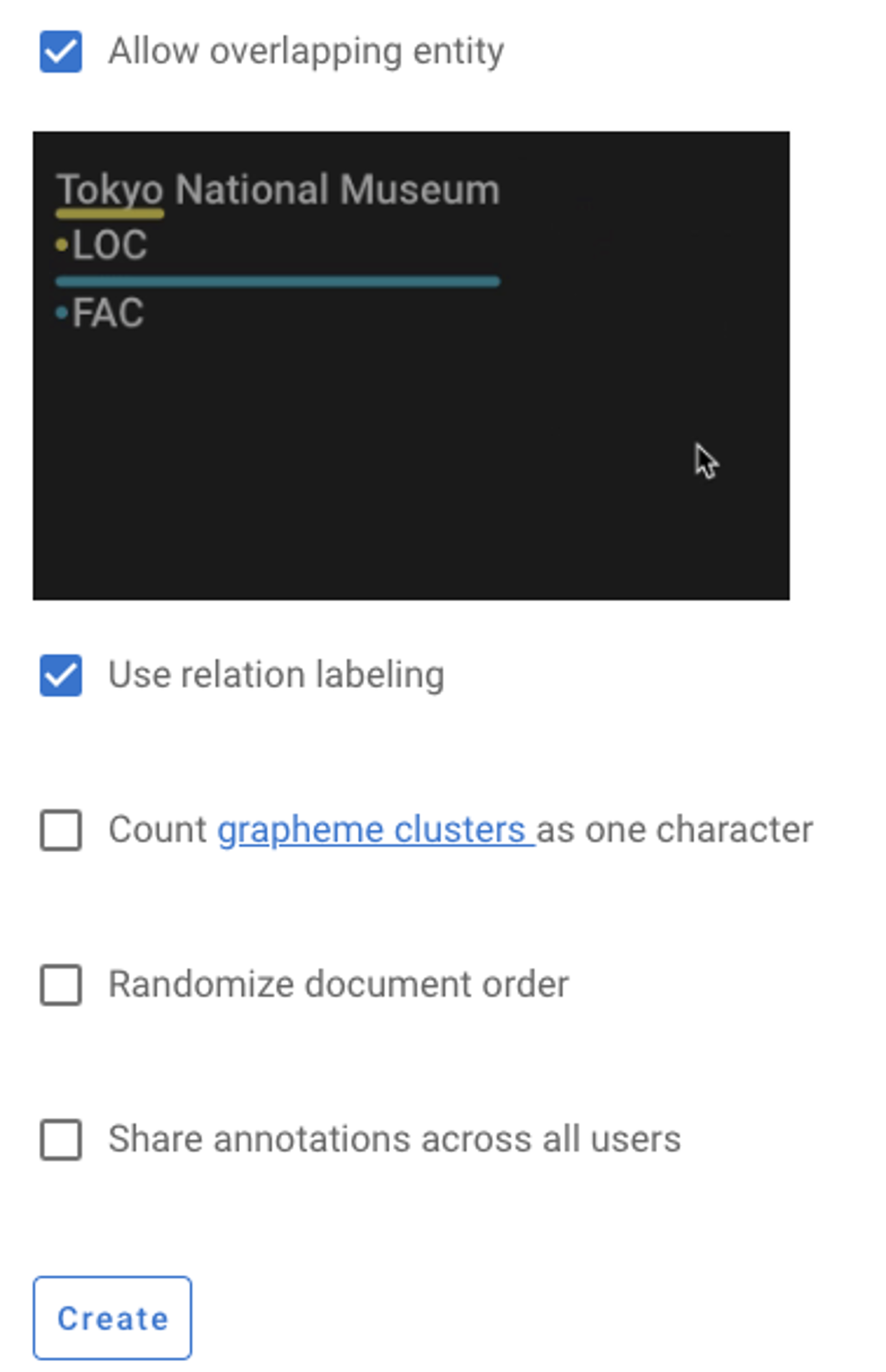• 设置标签。在Labels一栏点击`Actions``Create Label`手动设置或者`Import Labels`从文件导入。

• 最上边Span表示实体标签，Relation表示关系标签，需要分别设置。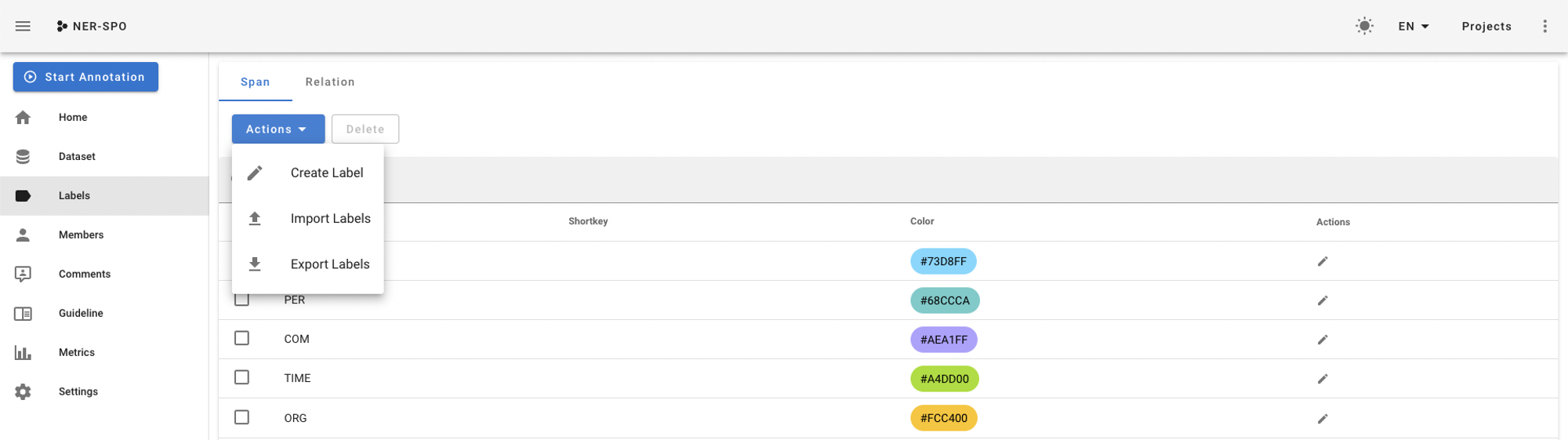• 导入数据。在Datasets一栏点击`Actions``Import Dataset`从文件导入文本数据。

• 根据文件格式（File format）给出的示例，选择适合的格式导入自定义数据文件。
• 导入成功后即跳转至数据列表。
• 标注数据。点击每条数据最右边的`Annotate`按钮开始标记。标记页面右侧的标签类型（Label Types）开关可在实体标签和关系标签之间切换。

• 实体标注：直接用鼠标选取文本即可标注实体。
• 关系标注：首先点击待标注的关系标签，接着依次点击相应的头尾实体可完成关系标注。
• 导出数据。在Datasets一栏点击`Actions``Export Dataset`导出已标注的数据。

### 将标注数据转化成UIE训练所需数据

• 将doccano平台的标注数据保存在`./data/`目录。对于语音报销工单信息抽取的场景，可以直接下载标注好的数据

### 各个任务标注文档

``! wget https://paddlenlp.bj.bcebos.com/datasets/erniekit/speech-cmd-analysis/audio-expense-account.jsonl! mv audio-expense-account.jsonl ./data/``

#### 可配置参数说明

• `doccano_file`: 从doccano导出的数据标注文件。
• `save_dir`: 训练数据的保存目录，默认存储在`data`目录下。
• `negative_ratio`: 最大负例比例，该参数只对抽取类型任务有效，适当构造负例可提升模型效果。负例数量和实际的标签数量有关，最大负例数量 = negative_ratio * 正例数量。该参数只对训练集有效，默认为5。为了保证评估指标的准确性，验证集和测试集默认构造全负例。
• `splits`: 划分数据集时训练集、验证集所占的比例。默认为[0.8, 0.1, 0.1]表示按照`8:1:1`的比例将数据划分为训练集、验证集和测试集。
• `task_type`: 选择任务类型，可选有抽取和分类两种类型的任务。
• `options`: 指定分类任务的类别标签，该参数只对分类类型任务有效。
• `prompt_prefix`: 声明分类任务的prompt前缀信息，该参数只对分类类型任务有效。
• `is_shuffle`: 是否对数据集进行随机打散，默认为True。
• `seed`: 随机种子，默认为1000.
``! python preprocess.py --input_file ./data/audio-expense-account.jsonl --save_dir ./data/ --negative_ratio 5 --splits 0.2 0.8 0.0 --seed 1000``

## 2.3 训练UIE模型

• 使用标注数据进行小样本训练，模型参数保存在`./checkpoint/`目录。

tips: 推荐使用GPU环境，否则可能会内存溢出。CPU环境下，可以修改model为`uie-tiny`，适当调下batch_size。

#### 可配置参数说明：

• `train_path`: 训练集文件路径。
• `dev_path`: 验证集文件路径。
• `save_dir`: 模型存储路径，默认为`./checkpoint`
• `learning_rate`: 学习率，默认为1e-5。
• `batch_size`: 批处理大小，请结合显存情况进行调整，若出现显存不足，请适当调低这一参数，默认为16。
• `max_seq_len`: 文本最大切分长度，输入超过最大长度时会对输入文本进行自动切分，默认为512。
• `num_epochs`: 训练轮数，默认为100。
• `model`: 选择模型，程序会基于选择的模型进行模型微调，可选有`uie-base``uie-tiny`，默认为`uie-base`
• `seed`: 随机种子，默认为1000.
• `logging_steps`: 日志打印的间隔steps数，默认10。
• `valid_steps`: evaluate的间隔steps数，默认100。
• `device`: 选用什么设备进行训练，可选cpu或gpu。
``! python finetune.py --train_path ./data/train.txt --dev_path ./data/dev.txt --save_dir ./checkpoint --model uie-tiny --learning_rate 1e-5 --batch_size 2 --max_seq_len 512 --num_epochs 50 --seed 1000 --logging_steps 10 --valid_steps 10``
``#! python finetune.py --train_path ./data/train.txt --dev_path ./data/dev.txt --save_dir ./checkpoint --model uie-base --learning_rate 1e-5 --batch_size 16 --max_seq_len 512 --num_epochs 50 --seed 1000 --logging_steps 10 --valid_steps 10``
• 使用小样本训练后的模型参数再次测试无法正确抽取的case。
``from paddlenlp import Taskflowschema = ['时间', '出发地', '目的地', '费用']few_ie = Taskflow('information_extraction', schema=schema, task_path='./checkpoint/model_best')few_ie(['10月16日高铁从杭州到上海南站车次d5414共48元',        '10月22日从公司到首都机场38元过路费'])``
``[{'时间': [{'text': '10月16日',    'start': 0,    'end': 6,    'probability': 0.9998620769863464}],  '出发地': [{'text': '杭州',    'start': 9,    'end': 11,    'probability': 0.997861665709749}],  '目的地': [{'text': '上海南站',    'start': 12,    'end': 16,    'probability': 0.9974161074329579}],  '费用': [{'text': '48',    'start': 24,    'end': 26,    'probability': 0.950222029031579}]}, {'时间': [{'text': '10月22日',    'start': 0,    'end': 6,    'probability': 0.9995716364718135}],  '目的地': [{'text': '首都机场',    'start': 10,    'end': 14,    'probability': 0.9984550308953608}],  '费用': [{'text': '38',    'start': 14,    'end': 16,    'probability': 0.9465688451171062}]}]``V1